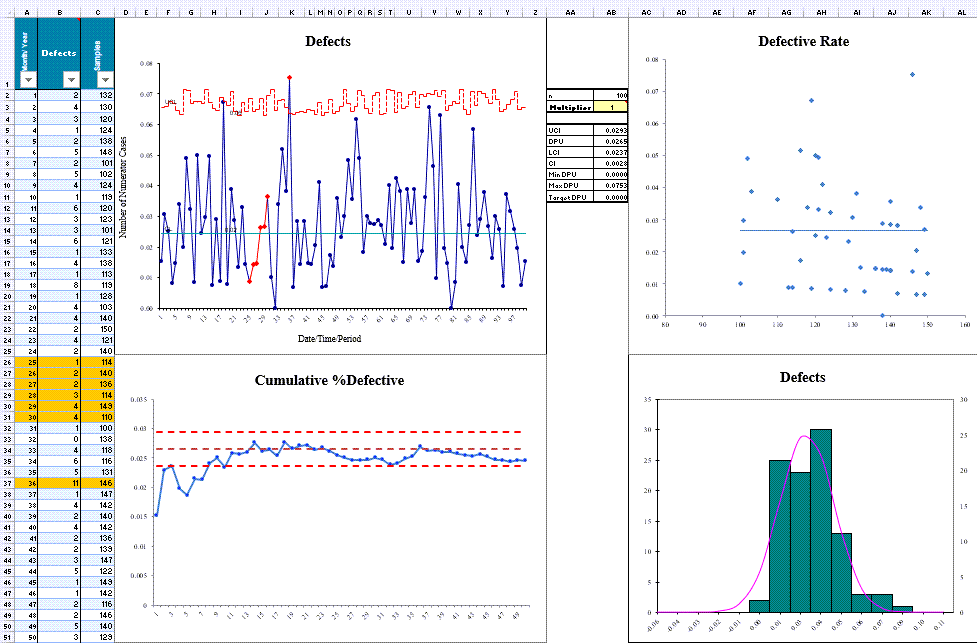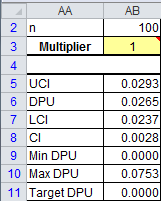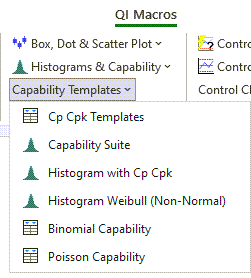# Need to do Poisson Capability Analysis But Don't Know How?

## QI Macros will do the calculations and analysis for you right in Excel!

### Example of QI Macros Poisson Capability Template### The Poisson Capability Template Consists of these Charts:

• u chart of defects to determine if the process is stable or not.
• chart of cumulative % defective
• the defective rate chart to determine if your data distribution is Poisson.
• histogram of defects

Tip: If your data is unstable and/or does not follow the Poisson Distribution, the calculated results may not be reliable.

### Example of Poisson Analysis Using the Output Shown Above

• The u chart is in control so capability analysis is possible. (Out of control data, as mentioned above, is not recommended)
• The Cumulative % Defective chart is also pretty stable.
• The Defective Rate chart shows points evenly distributed on either side of the average, so distribution is Poisson.
• If the Upper Confidence Interval (UCI) for DPU (cell AB:5) is greater than the target DPU (cell AB:11) , then you cannot be 95% confident that the process is capable.You can find this template by selecting QI Macros menu > Capability Templates > Poisson Capability.